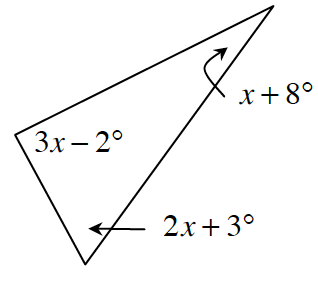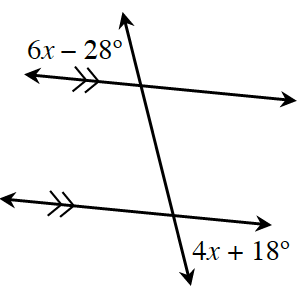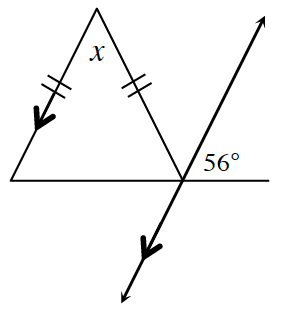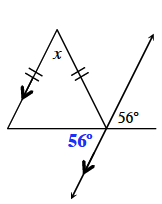### Home > GC > Chapter 2 > Lesson 2.3.3 > Problem2-121

2-121.

Use the relationships in the diagrams below to solve for $x$, if possible. If it is not possible, state how you know. If it is possible, justify your solution by stating which geometric relationships you use.

1.The angles in a triangle sum to $180º$.
So, $(3x-2º)+(2x+3º)+(x+8º)=180º$.

1.1.How can you use the parallel lines to find an angle within the triangle?